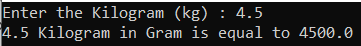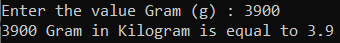# How to Convert Kilogram to Gram and Vice Versa in C#

## How to Convert Kilogram to Gram and Vice Versa in C# with Example

In this article, I am going to discuss How to Convert the Kilogram to Gram and Vice Versa in C# with Examples. Please read our previous article, where we discussed How to find the sum of odd numbers from 1 to N in C#. Here, in this article, first, we will discuss what is Kilogram and gram and then we will discuss how to convert Kilogram to Gram and Gram to Kilogram and finally, we will see how to convert Kilogram to Gram and Gram to Kilogram Program in C#.

1. Kilogram: The standard unit of measurement defined by the International System of Units (SI) to measure mass is called Kilogram. It is represented by the symbol ‘kg’.
2. Gram: A gram is also a unit of mass defined by the international system of units (SI). It is one-thousandth of a kilogram. It is denoted by the symbol ‘g’ and is used to measure light-weighted objects.
###### How to convert Kilograms to Grams

1 kilogram (kg) is equal to 1000 grams (g) i.e.1 kg = 1000 g
The mass m in grams (g) is equal to the mass m in kilograms (kg) times 1000: m(g) = m(kg) × 1000
Example: Convert 5kg to grams: m(g) = 5 kg × 1000 = 5000 g

###### How to convert Grams to Kilograms

1 gram (g) is equals to 1/1000 kilogram(kg) i.e. 1 g = 1/1000 kg
The mass m in kilograms (kg) is equal to the mass m in grams (g) time 1/1000: m(kg)=m(g) / 1000
Example: Convert 2000g to kilograms: m(kg) = 2000 / 1000 = 2 kg

##### Algorithm to Convert Kilograms to Grams

The following are the steps that we need to follow to solve this problem.
Step1: Take two variables named gram and kilogram.
Step2: Take the value of the kilogram from the user and store it into the kilogram variable.
Step3: Multiply the kilogram value with 1000 and store the result into the gram variable.
Step4: Print the value of gram as output.

##### Algorithm to Convert Grams to Kilograms

The following are the steps that we need to follow to solve this problem.
Step1: Take two variables named gram and kilogram.
Step2: Take the value of gram from the user and store it into the gram variable.
Step3: Divide the gram value by 1000 and store the result into the kilogram variable.
Step4: Print the value of the kilogram as output.

##### Example: C# Program to Convert Kilogram to Grams

The following C# Program will convert Kilogram to Grams.

```using System;
namespace DotNetTutorials
{
public class KilogramToGram
{
static void Main(string[] args)
{
Console.Write("Enter the Kilogram (kg) : ");
decimal gram = kilogram * 1000;
Console.WriteLine(\$"{kilogram} Kilogram in Gram is equal to {gram}");
}
}
}
```
###### Output:##### Example: C# Program to Convert Grams to Kilograms

The following C# Program will convert Grams to Kilograms.

```using System;
namespace DotNetTutorials
{
public class KilogramToGram
{
static void Main(string[] args)
{
Console.Write("Enter the value Gram (g) : ");
decimal kilogram = gram / 1000;
Console.WriteLine(\$"{gram} Gram in Kilogram is equal to {kilogram}");
}
}
}
```
###### Output:In the next article, I am going to discuss How to Find all the factors of a given number in C# with Examples. Here, in this article, I try to explain How to Convert a Kilogram to Gram and Vice Versa in C# with Examples and I hope you enjoy this article.

### 1 thought on “How to Convert Kilogram to Gram and Vice Versa in C#”

1.